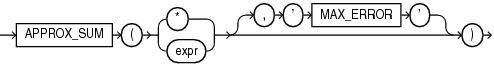## APPROX_SUM

SyntaxDescription of the illustration approx_sum.eps

Purpose

`APPROX_SUM` returns the approximate sum of an expression. If you supply `MAX_ERROR` as the second argument, then the function returns the maximum error between the actual and approximate sum.

You must use this function with a corresponding `APPROX_RANK` function in the `HAVING` clause. If a query uses `APPROX_COUNT`, `APPROX_SUM`, or `APPROX_RANK`, then the query must not use any other aggregation functions.

Note that `APPROX_SUM` returns an error when the input is a negative number.

Examples

The following query returns the 10 job types within every department that have the highest aggregate salary:

``````SELECT department_id, job_id,
APPROX_SUM(salary)
FROM   employees
GROUP BY department_id, job_id
HAVING
APPROX_RANK (
PARTITION BY department_id
ORDER BY APPROX_SUM(salary)
DESC ) <= 10;
``````